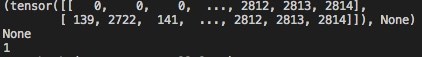# TypeError: index_select() received an invalid combination of arguments - got (Tensor, int, NoneType), but expected one of: * (Tensor input, name dim, Tensor index, *, Tensor out)

Hi everyone, I am trying to train a graph neural network on a PPI dataset. The code below shows a part of a code that passes messages from neighboring nodes through connecting edges. I get Type error as shown in the image below when I run this code through an import from the main program. From the error message, the error is from line 83, specifically "edge_index[idx] which returns a “none” instead of an element corresponding to an idx value in the array. But when I print the “idx”, it returns “1” so it should probably return index 1 element from the edge_index array but returns a “none” instead which throws an error. See attached the images.What were the `print` statements returning in the iteration before this error is raised?
Did you make sure that `edge_index[idx]` returned a valid tensor? If so, could you post an executable code snippet to reproduce this issue?

@ptrblck, the results for the print statements are shown in the image below. The tensor is the edge_index tuple with size “none”, “idx” prints “1” and edge_index[idx] prints a “none”.
With the posting of executable code to reproduce the issue, that will be a lot of code files to post because I’m running the main program which import a lot of different python files to execute including this message passing code. Probably I can post the full message passing code so that you can have a look.Here is the full message passing code:

import inspect
import torch
from torch_geometric.utils import scatter_

class MessagePassing(torch.nn.Module):
super(MessagePassing, self).init()

``````    self.aggr = aggr
assert self.aggr in ['add', 'mean', 'max']

self.flow = flow
assert self.flow in ['source_to_target', 'target_to_source']

self.message_args = inspect.getargspec(self.message)[1:]
self.update_args = inspect.getargspec(self.update)[2:]

def propagate(self, edge_index, size=None, **kwargs):
r"""The initial call to start propagating messages.

Args:
edge_index (Tensor): The indices of a general (sparse) assignment
matrix with shape :obj:`[N, M]` (can be directed or
undirected).
size (list or tuple, optional): The size :obj:`[N, M]` of the
assignment matrix. If set to :obj:`None`, the size is tried to
get automatically inferrred. (default: :obj:`None`)
**kwargs: Any additional data which is needed to construct messages
and to update node embeddings.
"""

size = [None, None] if size is None else list(size)
assert len(size) == 2

kwargs['edge_index'] = edge_index
i, j = (0, 1) if self.flow == 'target_to_source' else (1, 0)
ij = {"_i": i, "_j": j}

message_args = []
for arg in self.message_args:
if arg[-2:] in ij.keys():
tmp = kwargs[arg[:-2]]
if tmp is None:
message_args.append(tmp)
else:
idx = ij[arg[-2:]]
if isinstance(tmp, tuple):
assert len(tmp) == 2
if size[1 - idx] is None:
size[1 - idx] = tmp[1 - idx].size(0)
tmp = tmp[idx]

if size[idx] is None:
size[idx] = tmp.size(0)
#print(edge_index)
#print(edge_index[idx])
#print(idx)
tmp = torch.index_select(tmp, 0, edge_index[idx])
message_args.append(tmp)
else:
message_args.append(kwargs[arg])

size = size if size is None else size
size = size if size is None else size

kwargs['size'] = size
update_args = [kwargs[arg] for arg in self.update_args]

out = self.message(*message_args)
if self.aggr in ["add", "mean", "max"]:
out = scatter_(self.aggr, out, edge_index[i], dim_size=size[i])
else:
pass
out = self.update(out, *update_args)

return out

def message(self, x_j):  # pragma: no cover
return x_j

def update(self, aggr_out):  # pragma: no cover
return aggr_out``````

Based on the posted outputs it seems `edge_index` is a `tuple` containing a tensor and `None`.
If `idx` is set to `1`, you would index the `tuple` and get the `None` value as the return value.
I’m not sure what the `None` represents, but in case you want to index the stored tensor, you would have to use `edge_index[idx]`.

After changing it to edge_index[idx] which I agree, I got an error which says “Index tensor must have the same number of dimensions as self tensor” as shown in the image. From the printed output, the edge_index[idx] which is the index in the index_select module returns a flattened tensor, whilst that of the edge_index tensor is a tuple with a “tensor” and size “none”, thus throwing an error that the two tensors have unequal dimensions. The None represents the size the of the edge_index tensor. You can probably have a look at the full message passing code I attached in my previous message to see how the edge_index tensor was created.